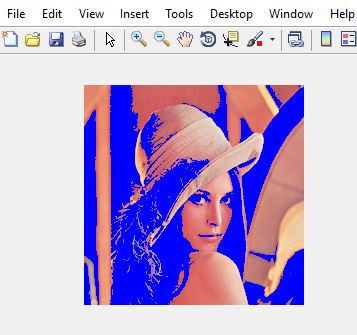# MATLAB | Change the color of background pixels by OTSU Thresholding

MATLAB also called Matrix Laboratory is a numerical computing environment and a platform for programming language. it was designed and developed by MathWorks. MATLAB is a framework that allows you to perform matrix manipulations, implementing algorithms, plotting functions and data, creating user-interfaces and interfacing with programs that are written in different programming languages i.e. C, C++, Python, Java etc..

OTSU Thresholding : OTSU thresholding is a segmentation algorithm through which we can segment an image into two or more than two regions. This algorithm checks on every single threshold if it can separate the two kinds of pixels optimally.

By OTSU thresholding we separate the foreground and background pixels.

Apprroach:

1. Read the image using imread() function and convert it to gray image using rgb2gray() function.
2. Graythresh the image using greythresh() function.
3. Detect the foreground and background pixels of the image.
4. Pick the background pixels and color them blue.

Below is the implementation of above approach –

 `% read the rgb image. ` `I = imread(``'leena.png'``);   ` ` `  `% convert it to gray image using rgb2gray() function.   ` `Ig = rgb2gray(I);     ` ` `  `% apply inbuilt otsu thresholding and ` `% convert the image to binary image.        ` `T = graythresh(Ig);          ` `Tg = T*255;   ` ` `  `% detect foreground and background ` `% pixels of the binary image.                ` `m = Ig > Tg                    ` `figure, imshow(m)   ` `        `  `I1 = I(:, :, 1);               ` `I2 = I(:, :, 2); ` `I3 = I(:, :, 3); ` `I1(~m) = 0; ` `I2(~m) = 0; ` ` `  `% in background pixels, take the third  ` `% channel and color it blue. ` `I3(~m) = 255;                  ` `In=cat(3, I1, I2, I3); ` `figure, imshow(In); `

Input Image:Output:My Personal Notes arrow_drop_upCheck out this Author's contributed articles.

If you like GeeksforGeeks and would like to contribute, you can also write an article using contribute.geeksforgeeks.org or mail your article to contribute@geeksforgeeks.org. See your article appearing on the GeeksforGeeks main page and help other Geeks.

Please Improve this article if you find anything incorrect by clicking on the "Improve Article" button below.

Article Tags :

Be the First to upvote.

Please write to us at contribute@geeksforgeeks.org to report any issue with the above content.